# 【万里征程——Windows App开发】绘制图形

2015/09/08 21:46

Rectangle

<Rectangle Fill="Yellow" Width="300" Height="200" Stroke="Blue" StrokeThickness="10" RadiusX="80" RadiusY="40"/>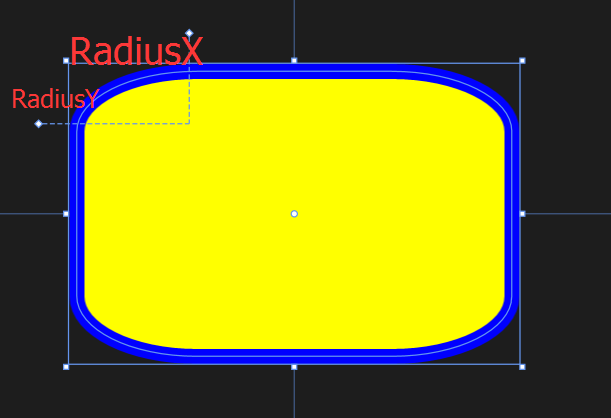Ellipse

<Ellipse Fill="Blue" Height="200" Width="350"/>

Polygon
Polygon则显得比较自由，只需要定义出各个顶点，它就会将这些点连接起来。那么我们可能会有疑问，需不需要确定图形的起始点和终点呢？答案是不用的，因为Polygon会自动将终点和起始点连接起来（它会假设图形是闭合的）。

<Polygon Fill="Green" Points="0,0,100,0,100,100,0,100 "/>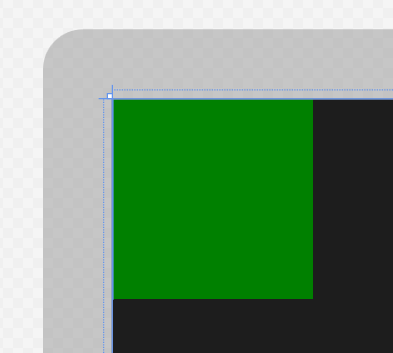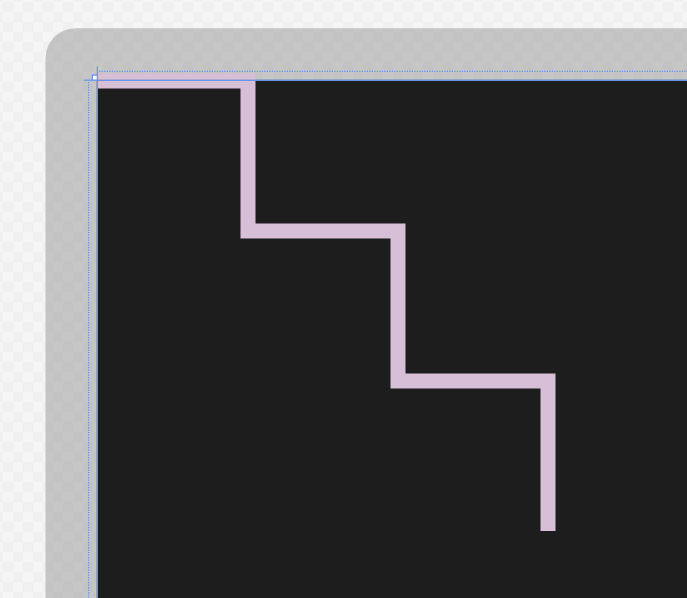Line Stroke="Red" StrokeThickness="10" X1="100" Y1="0" Y2="400" X2="400"/>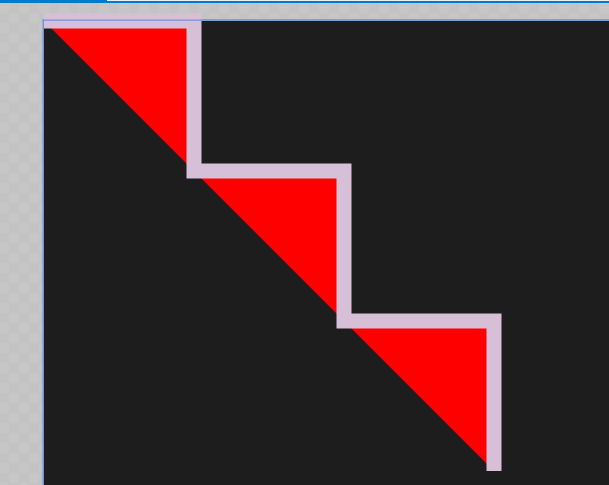Line
Line的使用也比较简单，但有一点要注意，必须设置好Stroke和StrokeThickness的属性值，否则Line就不会显示出来。原因很简单，因为它是直线。

<Line Stroke="Red" StrokeThickness="10" X1="100" Y1="0" Y2="400" X2="400"/>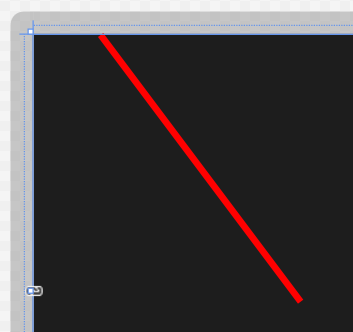Path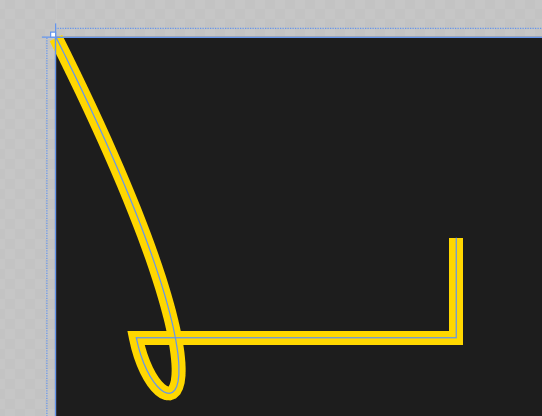<Path Stroke="Gold" StrokeThickness="7" Data="M 0,0 C 100,200 50,200 40,150 H 200 V 100 "/>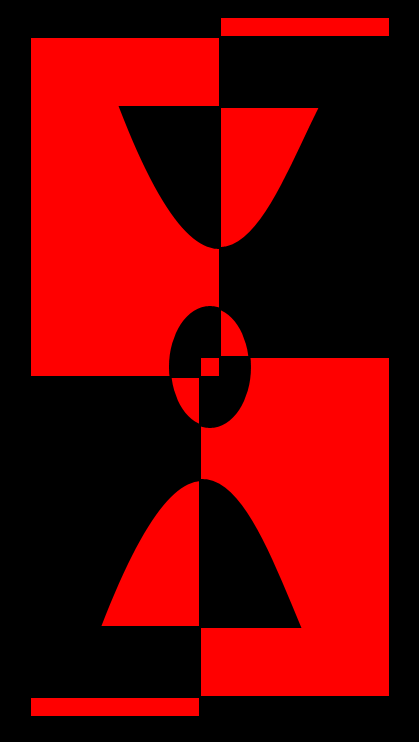<Path Stroke="Black" StrokeThickness="1" Fill="red">
<Path.Data>
<GeometryGroup>
<RectangleGeometry Rect="5,5 180,10" />
<RectangleGeometry Rect="5,5 95,180" />
<RectangleGeometry Rect="90,175 95,180"/>
<RectangleGeometry Rect="5,345 180,10" />
<PathGeometry>
<PathGeometry.Figures>
<PathFigureCollection>
<PathFigure IsClosed="true" StartPoint="50,50">
<PathFigure.Segments>
<PathSegmentCollection>
<BezierSegment Point1="100,180" Point2="125,100" Point3="150,50"/>
</PathSegmentCollection>
</PathFigure.Segments>
</PathFigure>
<PathFigure IsClosed="true" StartPoint="40,310">
<PathFigure.Segments>
<PathSegmentCollection>
<BezierSegment Point1="90,180" Point2="115,250" Point3="140,310"/>
</PathSegmentCollection>
</PathFigure.Segments>
</PathFigure>
</PathFigureCollection>
</PathGeometry.Figures>
</PathGeometry>
</GeometryGroup>
</Path.Data>
</Path>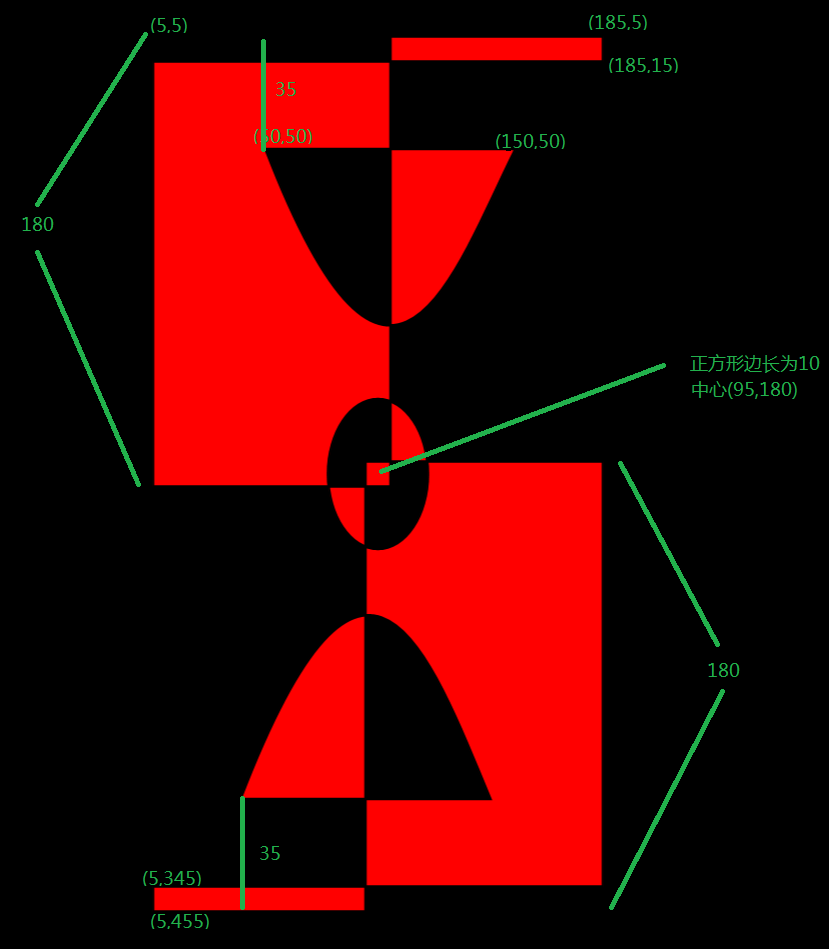http://blog.csdn.net/nomasp

0
0 收藏

### 作者的其它热门文章0 评论
0 收藏
0